×

Search anything:

# Merge Insertion Sort

#### Algorithms Sorting AlgorithmsIndian Technical Authorship Contest starts on 1st July 2023. Stay tuned.

Contents:

• Introduction
• Insertion Sort
• Merge Sort
• Combined Algorithm
• How it works
• Implementation

## INTRODUCTION:

Merge Insertion Sort is the combination of Merge sort and Insertion sort that minimizes the worst case time complexity for smaller value of n. So, in this article I will be giving a breif description about both Insertion sort and Merge sort and then, I will discuss how insertion sort and merge sort combine to develop a sorting algorithm, which has a better space and time complexity.

## INSERTION SORT:

In Insertion Sort we virtually split the given array into sorted and unsorted part. After that, we insert each element from the unsorted part to its correct position in the sorted part.
for example: If we want to sort this array: [5,4,3,2,4]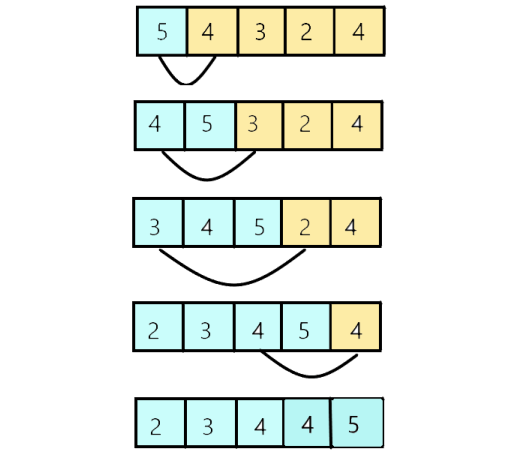Time Complexity:

• The worst case time complexity for sorting an array using insertion sort algorithm will be O(n^2), where n is total number of elements in the given array.
• The worst case time complexity of Insertion Sort is maximum among all sorting
algorithm but it takes least time if the array is already sorted i.e, its best
case time complexity is minimum which is O(n).

Space Complexity: O(1)

## MERGE SORT:

In Merge sort we split the given array in two parts and sort them individually and then we merges the both sorted halves. It is based on divide and conquer algorithm.
we divide the the given array into two equal halves untill only single element is left i.e, the array cannot be divide further.

Let's take an example to sort the given array = [8,3,4,12,5,6] using merge sort:

1. we divide the given array like this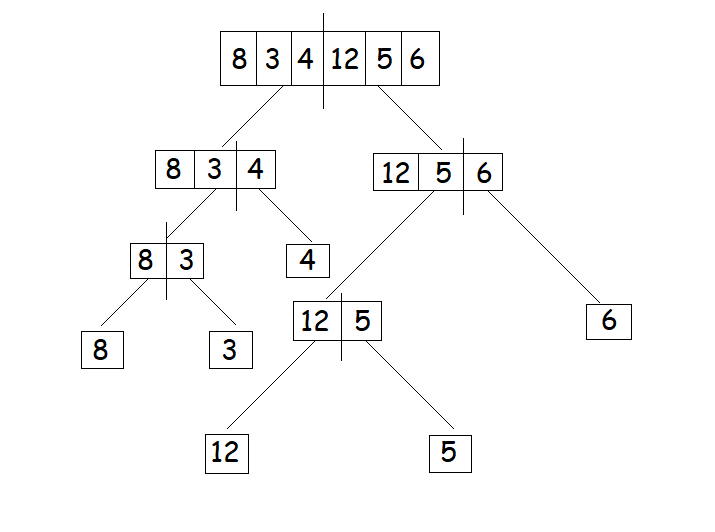2. Then, we will merge the the sorted parts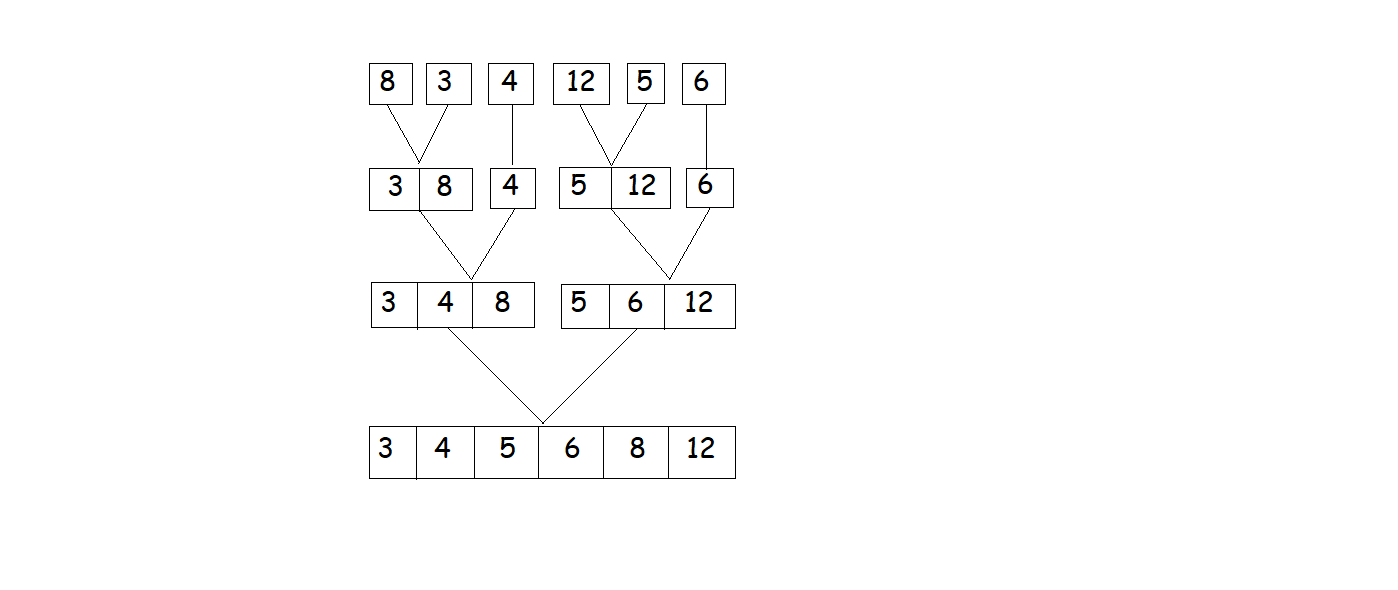Time Complexity:
The worst case, best case, and the average case time complexity of merge sort is O(N*log(N)). The worst case time complexity of merge sort is minumim among all sprting algothims.

Space Complexity: O(N)

## COMBINED ALGORITHM:

In 1959 Ford and Johnson initially described Merge Insertion Sort. So, it is also reffered as Ford and Johnson algorithm. Merge Insertion sort is basically the combination of Insertion sort and Merge sort or we can say Merge Insertion sort was formed as a resultant after combining the advantages of both the sorting techniques.

This sorting technique combines merging (like in merge-sort) and binary-search-insertion (like in insertion-sort), but, it is able to achieve better worst-case performance by selecting which elements to compare, as a result of this it will maximize the efficiency and decreases the time compleity of algorithm.

## HOW IT WORKS:

Now I am going to explain how merge sort works. Let's consider an array of size N.

1. First of all we will divide the given N elements of array into (N/K) groups of each group of size K.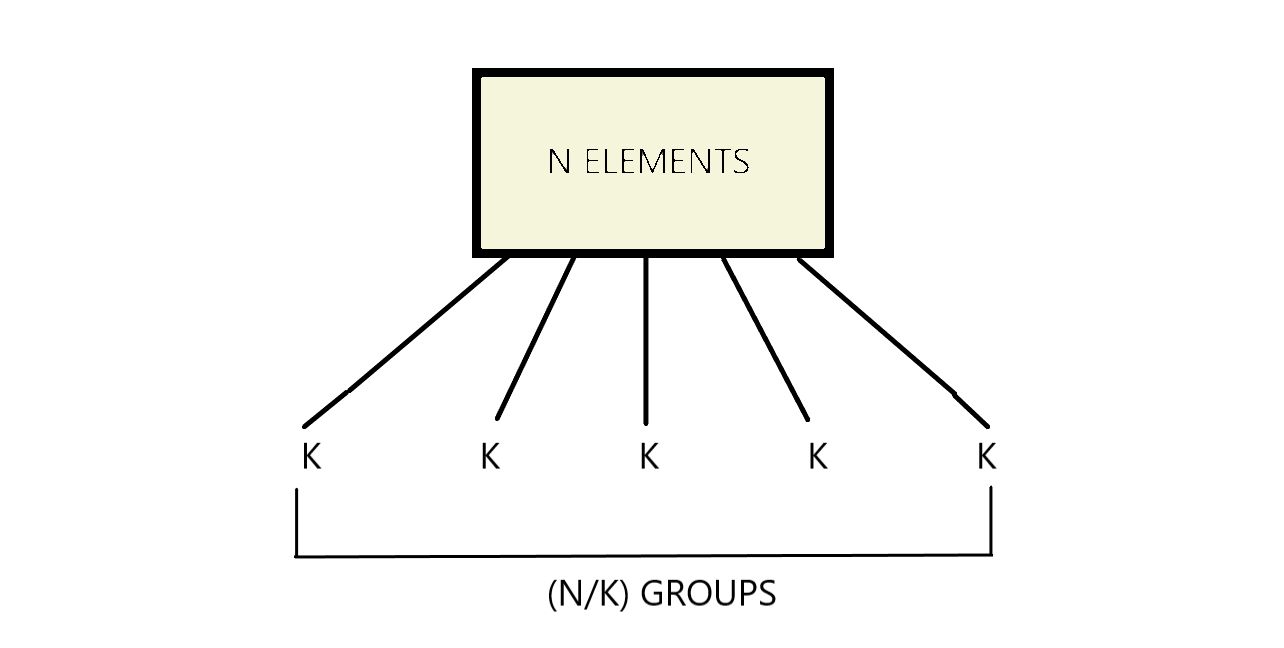2. Now we will sort each sub-array of size k like we used to do in Insertion sort. The time complexity for sorting each sub-array has been discussed below:

• As discussed earlier, the best case time complexity of Insertion Sort is O(N)
Since, In this case we have (N/K) sub-arrays each of size k, therefore the complexity of each sub-array will be O(k) and for whole array it will be
K*(N/K) i.e, O(N).
• Similarly, for the worst case, the time complexity for sorting each sub-array will be O(K^2) and for the whole array it will be (KK)(N/K) i.e, O(N*K).
3. Now after sorting each sub-array we will merge (N/K) sorted aub-arrays like we
used to do in merge sort.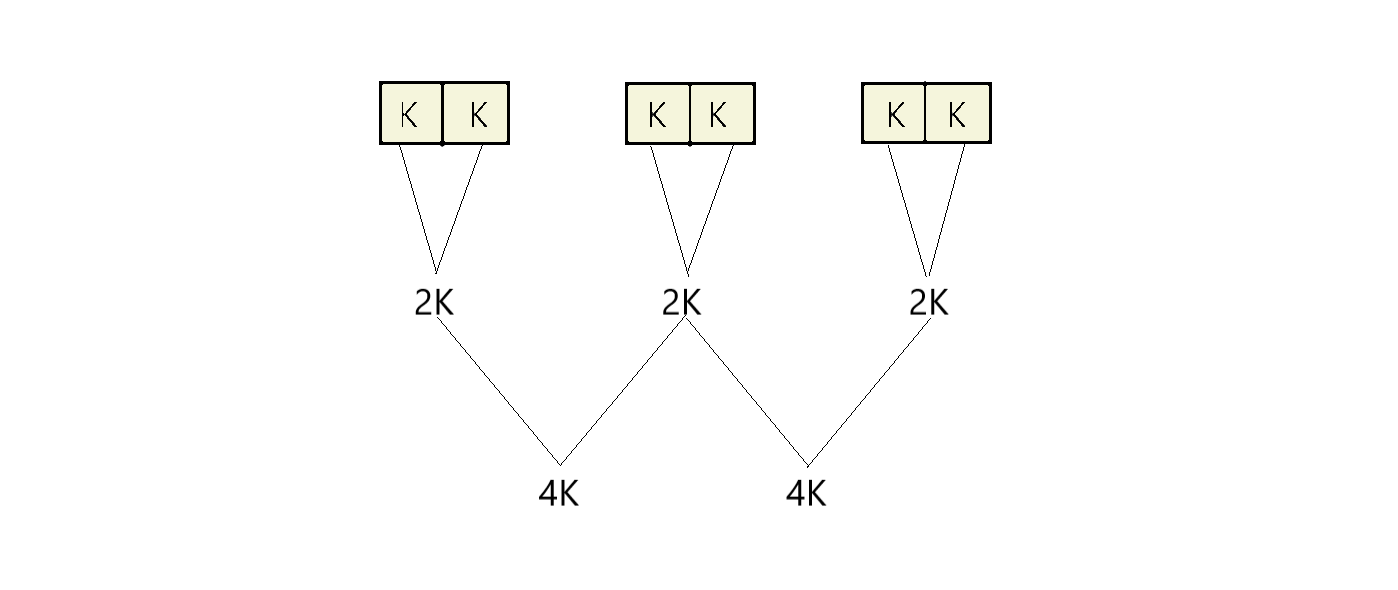#### TIME COMPLEXITY ANALYSIS:

Suppose we have to do i iterations in merge sort to merge the sorted parts:
(2^i) * K = N => (2^i) = N/K => i*(log(2)) = log(N/K) => i = log(N/K)
Therefore, Time required to merge = O(N*log(N/K))

When will best case occur:
Consider an array which is already sorted. so, the time complexity of sorting all the sub-arrays using insertion sort will be O(N), where N is the size of the given input array.
Therefore, the total time complexity of the combined algorithm in best case is:
Best Case: N+N*log(N/K)

When will worst case occur:
As discussed earlier the time complexity of sorting all the sub-arrays using insertion sort in worst case will be O(NK), where N is the size of the given input array.
Therefore, the total time complexity of the combined algorithm in worst case is:
Worst Case: N
K + N*log(N/K)

Space Complexity: O(1)

## IMPLEMENTATION:

``````public static final int K = 5;
public static void insertionSort(int A[], int p, int q) {
for (int i = p; i < q; i++) {
int tempVal = A[i + 1];
int j = i + 1;
while (j > p && A[j - 1] > tempVal) {
A[j] = A[j - 1];
j--;
}
A[j] = tempVal;
}
int[] temp = Arrays.copyOfRange(A, p, q +1);
Arrays.stream(temp).forEach(i -> System.out.print(i + " "));
System.out.println();
}

public static void merge(int A[], int p, int q, int r) {
int n1 = q - p + 1;
int n2 = r - q;
int[] LA = Arrays.copyOfRange(A, p, q +1);
int[] RA = Arrays.copyOfRange(A, q+1, r +1);
int RIDX = 0;
int LIDX = 0;
for (int i = p; i < r - p + 1; i++) {
if (RIDX == n2) {
A[i] = LA[LIDX];
LIDX++;
} else if (LIDX == n1) {
A[i] = RA[RIDX];
RIDX++;
} else if (RA[RIDX] > LA[LIDX]) {
A[i] = LA[LIDX];
LIDX++;
} else {
A[i] = RA[RIDX];
RIDX++;
}
}
}

public static void sort(int A[], int p, int r) {
if (r - p > K) {
int q = (p + r) / 2;
sort(A, p, q);
sort(A, q + 1, r);
merge(A, p, q, r);
} else {
insertionSort(A, p, r);
}
}

public static void main(String string[]) {
int[] A = { 2, 5, 1, 6, 7, 3, 8, 4, 9 };
sort(A, 0, A.length - 1);
Arrays.stream(A).forEach(i -> System.out.print(i + " "));
}
``````

This combined algorithm is better than both the individual algorithm because if

• K = 1, the above algorithm will work as a merge sort, which is better in time complexity.
• If K = N, the above algorithm will work as an insertion sort, better in space complexity.

With this article at OpenGenus, you must have the complete idea of Merge Insertion Sort.

#### Uddeshya Raj

Uddeshya Raj is pursuing B. Tech in Computer Science from Chandigarh University. He is an Algorithm and Data Structure Developer, Intern at OpenGenus.